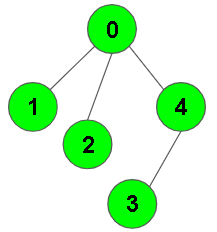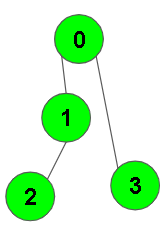XDAYS

:

HOUR

:

MINS

:

SEC

Copied to Clipboard
DFS of Graph
Easy Accuracy: 49.62% Submissions: 97677 Points: 2

Given a connected undirected graph. Perform a Depth First Traversal of the graph.
Note: Use recursive approach to find the DFS traversal of the graph starting from the 0th vertex from left to right according to the graph..

Example 1:

Input:Output: 0 1 2 4 3
Explanation:
0 is connected to 1, 2, 4.
1 is connected to 0.
2 is connected to 0.
3 is connected to 4.
4 is connected to 0, 3.
so starting from 0, it will go to 1 then 2
then 4, and then from 4 to 3.
Thus dfs will be 0 1 2 4 3.

Example 2:

Input:Output: 0 1 2 3
Explanation:
0 is connected to 1 , 3.
1 is connected to 2.
2 is connected to 1.
3 is connected to 0.
so starting from 0, it will go to 1 then 2
then back to 0 then 0 to 3
thus dfs will be 0 1 2 3.

You dont need to read input or print anything. Your task is to complete the function dfsOfGraph() which takes the integer V denoting the number of vertices and adjacency list as input parameters and returns  a list containing the DFS traversal of the graph starting from the 0th vertex from left to right according to the graph.

Expected Time Complexity: O(V + E)
Expected Auxiliary Space: O(V)

Constraints:
1 ≤ V, E ≤ 104

We are replacing the old Disqus forum with the new Discussions section given below.

Editorial

We strongly recommend solving this problem on your own before viewing its editorial. Do you still want to view the editorial?

My Submissions: